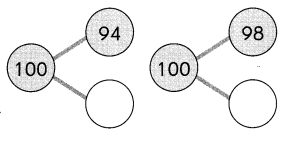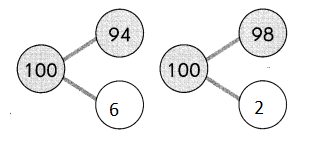ExampleQuestion 1.
26 + 96 = ?
26 + 100 = ___
26 + 96 = ___ – ___ – ___
= ____
So, 26 + 96 = ___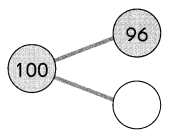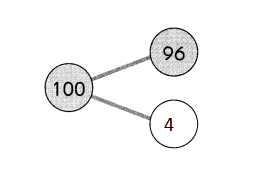26 + 100 = 126
26 + 96 = 126 – 4
= 122
So, 26 + 96 = 122

Question 2.
38 + 95 = ?
38 + 100 = ___
___ – ___ = ___
So, 38 + 95 = ___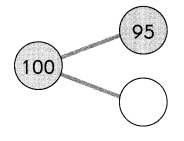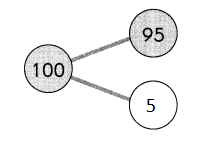38 + 100 = 138
138 – 5 = 133
So, 38 + 95 = 133

First, add the hundreds. Then, subtract the extra ones.

Example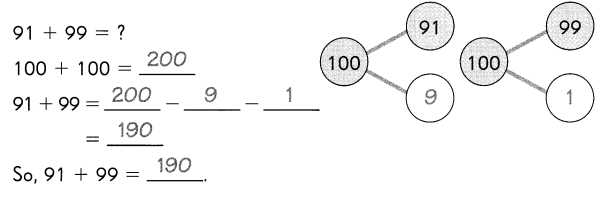Question 3.
98 + 96 = ?
100 + 100 = ___
98 + 96 = ___ – ___ – ___
= ___
So, 98 + 96 = ____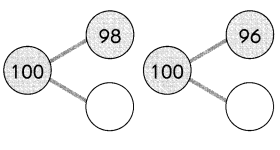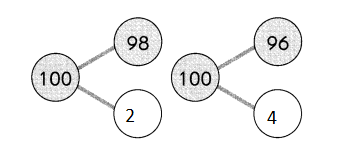100 + 100 = 200
98 + 96 = 200 – 2 – 4
= 194
So, 98 + 96 = 194

Question 4.
94 + 98 = ?
100 + 100 = ___
94 + 98 = ___ – ___ – ____
= ____
So, 94 + 98 = ___